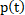## 4.1 Objective Test Methodologies for Assessment of Immersive Audio Systems in the Sending Direction

26.2603GPPObjective test methodologies for the evaluation of immersive audio systemsRelease 18TS

### 4.1.1 Diffuse-field Send Frequency Response for Scene-based Audio

#### 4.1.1.1 Introduction

This test is applicable to UEs capturing scene-based audio (e.g. First and Higher Order Ambisonics).

NOTE: Currently, the test method uses a periphonic loudspeaker array for generation of a diffuse-field. Additional loudspeaker setups for the derivation of the diffuse sound field are under consideration.

General test conditions

Free-field propagation conditions

– The test environment shall contain a free-field volume, wherein free-field sound propagation conditions shall be observed.

– The free-field sound propagation conditions shall be observed down to a frequency of 200 Hz or less.

– Qualification of the free-field volume shall be performed using the method and limits for deviation from ideal free-field conditions described in .

Test environment noise floor

Within the free-field volume, the equivalent continuous sound level of the test environment in each 1/3rd octave band, Leq(f), shall be less than the limits of the NR10 curve, following the noise rating determination procedures in .

#### 4.1.1.2 Definition

The Diffuse-field Send Frequency Response for Scene-based Audio is defined as the transfer function, , between: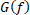, the estimated sound pressure magnitude spectrum obtained from a diffuse-field scene-based audio capture and reference synthesis at the geometric center of a free-field volume; and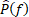, the sound pressure magnitude spectrum obtained from a diffuse-field microphone recording the same diffuse field at the origin of a spherical coordinate system.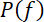Figure 1 describes a typical block diagram for the scene-based audio sending direction with measurement points when using a periphonic loudspeaker array.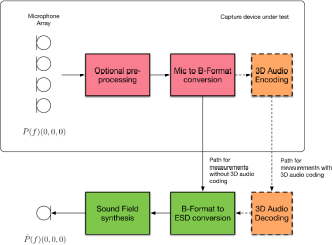Figure 1: Scene-based audio capture block diagram for sending direction measurements

Definition of Equivalent Spatial Domain

The equivalent spatial domain representation, w(t), of a Nth order Ambisonics soundfield representation c(t) is obtained by rendering c(t) to K virtual loudspeaker signals wj (t), 1 ≤ jK, with K = (N+1)2. The respective virtual loudspeaker positions are expressed by means of a spherical coordinate system, where each position lies on the unit sphere, i.e., a radius of 1. Hence, the positions can be equivalently expressed by order-dependent directions Ωj(N)=(θj(N), φ j(N)), 1 ≤ jK, where θj(N) and φ j(N) denote the inclinations and azimuths, respectively. These directions are defined according to  and reproduced in Annex A for convenience.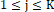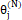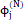The rendering of into the equivalent spatial domain can be formulated as a matrix multiplication: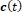w(t) = (Ψ(N,N))-1 ⋅c(t),

where (⋅)-1 denotes the inversion.

The matrix Ψ(N,N) of order N with respect to the order-dependent directions Ωj(N) is defined by:

Ψ(N,N) := [S1(N) S2(N) SK(N)],

with:

j(N) := [S00(Ωj(N)) S-1-1(Ωj(N)) S-10(Ωj(N)) S-11(Ωj(N)) S-11(Ωj(N)) … SNN(Ωj(N))]T ,where Snm(⋅) represents the real valued spherical harmonics of the order n and degree m as defined in .

The matrix Ψ(N,N) is invertible so that the HOA representation c(t) can be converted back from the equivalent spatial domain by:

c(t) = Ψ(N,N)·w(t)

#### 4.1.1.3 Test method with periphonic array

##### 4.1.1.3.1 Test Conditions

Periphonic loudspeaker array

a) A periphonic loudspeaker array shall be placed within the free-field volume with the geometric center of the periphonic loudspeaker array coinciding with the geometric center of the free-field volume.

b) The periphonic loudspeaker array shall have a radius greater or equal than 1 meter.

c) The periphonic loudspeaker array shall be composed of (N+1)2 coaxial loudspeaker elements. Each of the (N+1)2 coaxial loudspeaker elements shall be equalized (if necessary) and level compensated to conform with the operational room response curve limits given in  Section 8.3.4.1. N should be equal or greater than the maximum ambisonics order supported by the device under test (DUT), e.g. N>=4 for a DUT supporting 4th order Ambisonics capture.

d) The (N+1)2 coaxial loudspeaker elements shall be positioned according to the azimuth and elevation coordinates given in Annex B.

e) All coaxial loudspeaker elements shall be oriented such that their acoustic axis intersects at the geometric center of the free field volume.

f) The radius of each coaxial loudspeaker element shall be such that, at the geometric center of the free-field volume, the far field approximation for the coaxial loudspeaker axial pressure amplitude decay holds true.

##### 4.1.1.3.2 Measurement

Reference Spectrum measurement for periphonic loudspeaker array method

a) A diffuse-field / random incidence, or multi-field microphone is mounted in the free-field volume such that the tip of the microphone corresponds to the geometric center of the free-field volume and the geometric center of the periphonic loudspeaker array.

NOTE 1: Diffuse-field / random incidence microphones, are described in .

b) (N+1)2 decorrelated pink noise signals are played simultaneously over each of the (N+1)2 coaxial loudspeakers of the periphonic loudspeaker array.

c) The playback level is adjusted such that the LAeq, measured over a 30s time window at the geometric center of the periphonic loudspeaker array, is equal to 78dBSPL(A) ± 0.5dB.

d) The reference sound pressure at the geometric center of the free-field volume, p(t), is captured with the diffuse-field or multi-field microphone.

e) The magnitude spectrum of the reference sound pressure, P(f), is calculated for the 1/12th octave intervals as given by the R40 series of preferred numbers in .

NOTE 2: For ideal (calibrated) loudspeakers, the P(f) spectra should have equal energy in each 1/12th octave intervals.

Estimated Spectrum measurement

a) The scene-based audio capture device under test is mounted in the free-field volume such that its geometric center coincides with the geometric center of free-field volume and the geometric center of the periphonic loudspeaker array.

b) (N+1)2 decorrelated pink noise signals are played simultaneously over each of the (N+1)2 coaxial loudspeakers of the periphonic loudspeaker array. The pink noise signals shall be identical to the signals used for the reference spectrum measurement.

c) The B-format scene-based audio format representation (compressed or uncompressed, depending on the use case being tested) is stored for offline analysis.

d) The B-format scene-based audio format representation is uncompressed (if necessary) and converted to an equivalent spatial domain representation of order NDUT (B-Format to ESD conversion in Figure 1), where NDUT corresponds to the Ambisonics order of the device under test.

e) , the estimate of the sound field at the geometric center of the free-field volume and periphonic loudspeaker array, is synthesized using the equivalent spatial domain representation of order NDUT.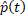NOTE 3: can be taken from the W component of the B-Format signal, as an alternative to implementing the B-Format to ESD conversion in step d).f) The magnitude spectrum of the estimated sound pressure, , is calculated for the 1/12th octave intervals as given by the R40 series of preferred numbers in .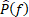Calculation of send frequency response for scene-based audio

The send frequency response for scene-based audio, G(f), is calculated as .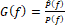#### 4.1.1.4 Test method with loudspeaker array and turn table

##### 4.1.1.4.1 Test Conditions

Loudspeaker array

a) A calibrated loudspeaker array shall be placed within the free-field volume.

b) The loudspeaker array shall comprise one or several semi-arcs having a radius greater or equal than 1 meter. The radius shall be reported.

c) The loudspeaker array shall be composed of N+1 loudspeaker elements. The ambisonic order N shall be reported.

d) Each loudspeaker in the array shall be calibrated with a frequency response of [at least 100 Hz-20,000 Hz] and minimum phase response.

e) The coordinates of the loudspeaker elements are defined according to a Gaussian spherical grid  of order N. Directions shall comply with Annex B.1 and the N+1 elevations of the spherical grid shall be reported.

Turn table

a) A turn table with a resolution of 0.5 degrees shall be used. The rotation axis of the turn table and the vertical axis of the semi-arcs shall be aligned The turn table shall be adjusted in height so that the device under test is positioned at the geometric center of the loudspeaker array.

b) For measurement, an azimuth step of 180/(N+1) degrees shall be used.

##### 4.1.1.4.2 Measurement

Reference Spectrum measurement

a) A diffuse-field / random incidence, or multi-field microphone is mounted in the free-field volume such that the tip of the microphone corresponds to the geometric center of the free-field volume and the geometric center of the loudspeaker array.

NOTE 1: Diffuse-field / random incidence microphones, are described in .

Repeat steps b-c) with an azimuth angular resolution of 180/(N+1) degrees:

b) An exponential sweep sine signal is played over each of the N+1 loudspeakers of the loudspeaker array.

c) The impulse response at the geometric center of the loudspeaker array is measured for each loudspeaker position.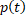d) The magnitude spectrum of the reference sound pressure, P(f), is calculated for the 1/12th octave intervals as given by the R40 series of preferred numbers in .

NOTE 2: For ideal (calibrated) loudspeakers, the P(f) spectra should have equal energy in each 1/12th octave intervals.

Estimated Spectrum measurement

a) The scene-based audio capture device under test is mounted in the free-field volume such that its geometric center coincides with the geometric center of free-field volume and the geometric center of the loudspeaker array.

b) Repeat steps b-c) with an azimuth angular resolution of 180/(N+1) degrees::

c) An exponential sweep sine signal is played over each of the N+1 loudspeakers of the loudspeaker array. The sweep signals shall be identical to the signals used for the reference spectrum measurement.

d) The impulse response at the geometric center of the loudspeaker array is measured for each loudspeaker position.e) The magnitude spectrum of the estimated sound pressure, , is calculated for the 1/12th octave intervals as given by the R40 series of preferred numbers in .Calculation of send frequency response for scene-based audio

The send frequency response for scene-based audio, G(f), is calculated as .Due to practical constraints (e.g. reflections on turn table), measurements for specific elevations (e.g. < – degrees) may be unreliable and discarded. In this case, the above measurement procedure may be conducted in two phases by measuring only directions for one hemisphere (e.g. top hemisphere, with elevations >0) in each phase. The device under test shall be flipped upside down between the two phases, and this two-phase approach shall be reported.

### 4.1.2 Directional response measurement for scene-based audio

#### 4.1.2.1 Definition

The directional response for scene-based audio is defined as the transfer function, represented as an impulse response, h(iibetween a device under test and a loudspeaker located at an equal distance r and L predefined directions, (iii=1,…,L.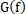#### 4.1.2.2 Test conditions

Free-field propagation conditions

– The test environment shall contain a free-field volume, wherein free-field sound propagation conditions shall be observed.

– The free-field sound propagation conditions shall be observed down to a frequency of 200Hz.

Test environment noise floor

The equivalent continuous sound level of the test environment in each 1/3rd octave band, Leq(f), shall be less than the limits of the NR10 curve, following the noise rating determination procedures in .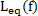Loudspeaker array

A real or simulated loudspeaker array comprising L loudspeakers located be a set of predefined directions (ii, i=1,…,L, from the geometric center of the loudspeaker array shall be used.

#### 4.1.2.3 Measurement

For each loudspeaker position (ii, i=1,…,L , the following procedure shall be used:

a) An exponential sweep sine test signal is played over the loudspeaker.

NOTE: The impact of codec on the exponential sweep sine test signal needs to be verified before performing the measurements. An activation signal may be needed.

b) The impulse response h(ii at the geometric center of the loudspeaker array is measured.